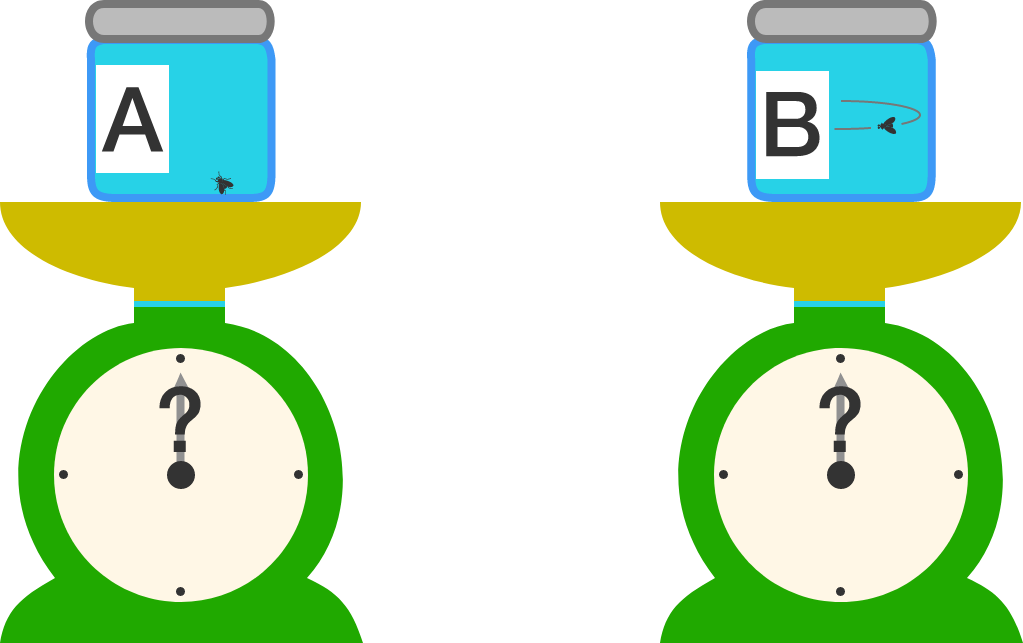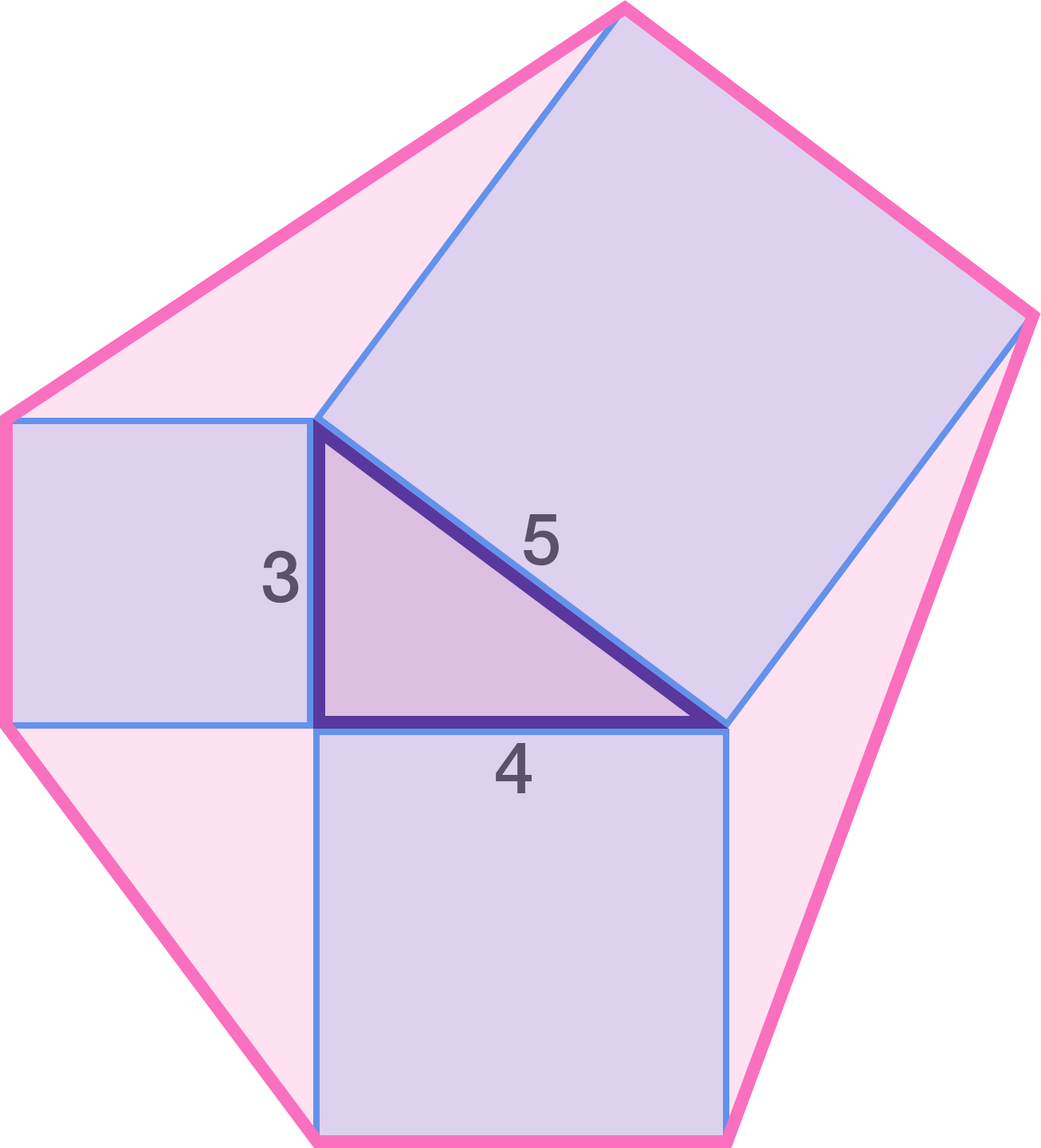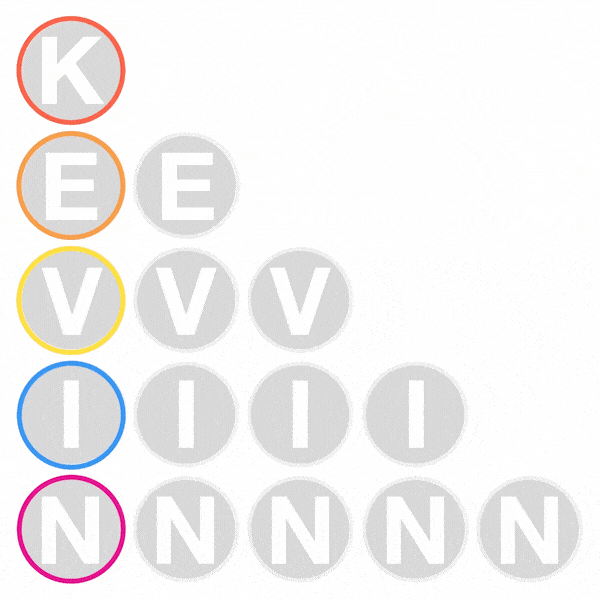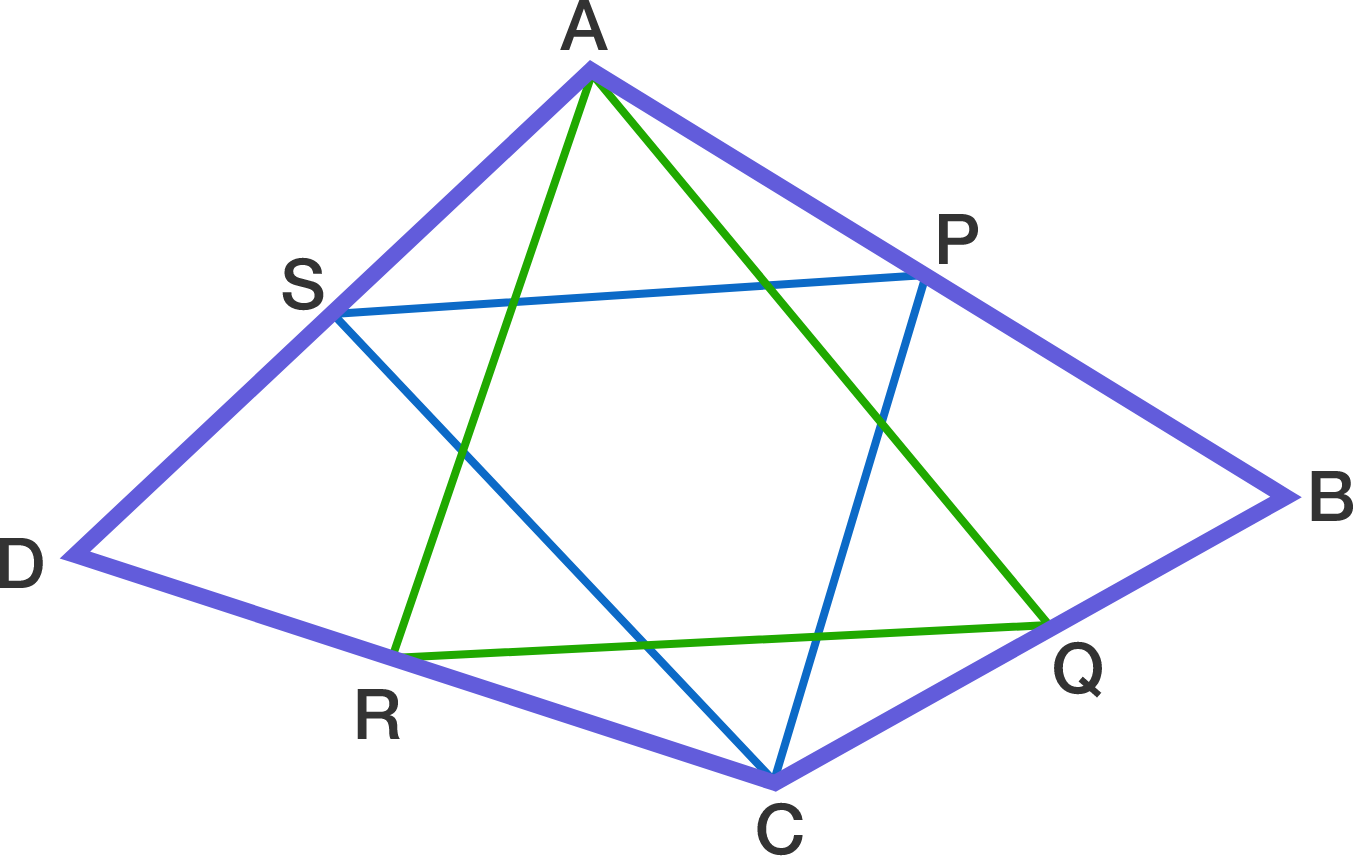# Problems of the Week

Contribute a problem

# 2017-03-27 IntermediateTwo identical jars $A$ and $B$ each contain a fruit fly of equal mass. The lids of the jars are tightly closed. In jar $A$, the fly is sitting; in jar $B$, the fly is flying at a constant speed in a horizontal circle.

The jars are placed on weighing scales to see which jar weighs more. Which jar will show a greater reading on the scale?

Amanda, Billy, Caleb, David, and Ellie are told that they are each given a distinct integer from 1 to 5 inclusive. They each know their own integer, but are not told the integer of anyone else. They make the following statements:

Amanda: "My number has an odd number of positive factors."
Billy: "Really? My number is either odd or prime, but not both."
Caleb: "I now know Amanda's number."

Given that David's number is less than Amanda's number, what number does Ellie have?Construct a square on each side of a triangle with side lengths 3, 4, and 5. Then, construct a hexagon by joining the vertices of the squares which are not vertices of the 3-4-5 triangle.

Find the area of this hexagon.You are given 15 letters above with which you can write my name KEVIN.
Suppose that, each time you go down one row, you have 3 choices: go straight down, or go diagonally one to the left, or go diagonally one to the right.

Then, how many ways are there to spell out KEVIN?

Bonus: Can you generalize to $n$ rows?Let ABCD be a convex quadrilateral. Also let P, Q, R, S be the respective midpoints of sides AB, BC, CD, DA such that triangles AQR and CSP are both equilateral.

Find the largest angle (in degrees) of quadrilateral ABCD.

×